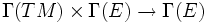# Affine space of connections

(diff) ← Older revision | Latest revision (diff) | Newer revision → (diff)
Given a differential manifold$M$ and a vector bundle$E$ over$M$, the affine space of connections on$M$ is defined as the set of all connections on$M$, viewed as a subset of the vector space of all bilinear maps:$\Gamma(TM) \times \Gamma(E) \to \Gamma(E)$